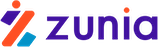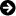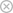Tuyển sinh 2023 dành cho Gen-Z# Mô hình chiết khấu

Chia sẻ: Diemanh Diemanh | Ngày: | Loại File: XLS | Số trang:14325
lượt xem
103

Mô hình chiết khấu FCFE

Chủ đề:

## Nội dung Text: Mô hình chiết khấu

1.  Two­Stage FCFE Discount Model Two-Stage FCFE Discount Model This model is designed to value the equity in a firm, with two stages of growth, an initial period of higher growth and a subsequent period of stable growth. Assumptions 1. The firm is expected to grow at a higher growth rate in the first period. 2. The growth rate will drop at the end of the first period to the stable growth rate. 3. The free cashflow to equity is the correct measure of expected cashflows to stockholders. The user has to define the following inputs: 1. Length of high growth period 2. Expected growth rate in earnings during the high growth period. 3. Capital Spending, Depreciation and Working Capital needs during the high growth period. 4. Expected growth rate in earnings during the stable growth period. 5. Inputs for the cost of equity. Page
2.  Two­Stage FCFE Discount Model Inputs to the model Current Earnings per share = $4.00 (in currency) Current Dividends per share =$0.25 (in currency) Current Capital Spending/sh = $3.70 (in currency) Current Depreciation / share =$1.70 (in currency) Current Revenues/ share = $20.00 Working Capital/ share =$8.00 (in currency) Chg. Working Capital/share = $1.00 Enter length of extraordinary growth period = 5 (in years) Do you want to enter cost of equity directly? No (Yes or No) If yes, enter the cost of equity = (in percent) If no, enter the inputs to the cost of equity Beta of the stock = 1.3 Riskfree rate= 7.00% (in percent) Risk Premium= 5.50% (in percent) Earnings Inputs Do you want to use the historical growth rate? Yes (Yes or No) If yes, enter EPS from five years ago =$0.49 (in currency) Do you have an outside estimate of growth ? Yes (Yes or No) If yes, enter the estimated growth: 19.00% (in percent) Do you want to calculate the growth rate from fundamentals? Yes (Yes or No) If yes, enter the following inputs: Net Income Currently = $1,077.00 (in currency) Interest Expense Currently =$53.85 Last year (in currency) Book Value of Debt = $600.00$550.00 (in currency) Book Value of Equity = $5,445.00$5,130.00 (in currency) Tax Rate on Income= 36.00% (in percent) Page
3.  Two­Stage FCFE Discount Model The following will be the inputs to the fundamental growth formulation: ROC = 19.57% D/E = 11.02% (in percent) Retention = 93.75% Interest Rate= 8.98% (in percent) Do you want to change any of these inputs for the high growth period? No (Yes or No) If yes, specify the values for these inputs (Please enter all variables) ROC = 19.57% D/E = 11.02% (in percent) Retention = 93.75% Interest Rate= 8.98% (in percent) Specify weights to be assigned to each of these growth rates: Historical Growth Rate = 10.00% (in percent) Outside Prediction of Growth = 40.00% (in percent) Fundamental Estimate of Growth = 50.00% (in percent) Enter growth rate in stable growth period? 6.00% (in percent) Beta Will the beta to change in the stable period? Yes (Yes or No) If yes, enter the beta for stable period = 1.1 Capital Spending, Depreciation & Working Capital Do you want all these items to grow at the same rate as earnings ? No (Yes or No) If not, enter the growth rates for each of the following items: Capital Spending Depreciation Revenues High Growth 20% 20% 18% (in percent) Stable Growth Do not enter Do not enter 6% (in percent) Do you want to keep the current fraction of working capital to revenues? Yes (Yes or No) Specify working capital as a percent of revenues: 40% (in percent) Do you want to use the current debt ratio as your desired mix? Yes (Yes or No) If no, enter the following inputs for financing mix, Desired debt financing proportion - Capital Spending (in percent) Page
4.  Two­Stage FCFE Discount Model Desired debt financing proportion - Working Capital (in percent) Capital spending and Depreciation during Stable Growth Aswath Damodaran: Is capital spending to be offset by depreciation in stable period? No If you are going to  (Yes or No) Aswath Damodaran: assume perpetual  Do you want to compute your reinvestment rate from fundamentals? Yes Yes or No. If yes, enter  growth, you should  the return on equity that  Return on equity in stable growth period 12% answer no here and  your firm will have in  make sure that cap ex  If no, enter capital expenditures as % of depreciation in stable growth 150% perpetuity below. If no,  (in percent) is higher than  enter cap ex as a  percent of depreciatiion. Page
5. If you are going to  Aswath Damodaran: assume perpetual  Yes or No. If yes, enter  growth, you should   Two­Stage FCFE Discount Model the return on equity that  answer no here and  your firm will have in  make sure that cap ex  perpetuity below. If no,  is higher than  enter cap ex as a  percent of depreciatiion. Output from the program Cost of Equity = 14.15% Proportion of Debt: Capital Spending (DR)= 9.93% Proportion of Debt: Working Capital (DR)= 9.93% Current Earnings per share= $4.00 Page 6. Two­Stage FCFE Discount Model (Capital Spending - Depreciation)*(1-DR)$1.80 Change in Working Capital * (1-DR) $0.90 Current FCFE$1.30 Growth Rate in Earnings per share Growth Rate Weight Historical Growth = 52.19% 10.00% Outside Estimates = 19.00% 40.00% Fundamental Growth = 19.77% 50.00% Weighted Average 22.71% Growth Rate in capital spending, depreciation and working capital High Growth Stable Growth Growth rate in capital spending = 20.00% Do not enter Growth rate in depreciation = 20.00% Do not enter Growth rate in revenues = 18.00% 6.00% Working Capital as percent of revenues = 40.00% (in percent) The FCFE for the high growth phase are shown below (upto 6 years) 1 2 3 4 Earnings $4.91$6.02 $7.39$9.07 - (CapEx-Depreciation)*(1-DR) $2.16$2.59 $3.11$3.74 -Chg. Working Capital*(1-DR) $1.30$1.53 $1.81$2.13 Free Cashflow to Equity $1.45$1.90 $2.47$3.20 Present Value $1.27$1.46 $1.66$1.89 Growth Rate in Stable Phase = 6.00% FCFE in Stable Phase = $5.90 Cost of Equity in Stable Phase = 13.05% Price at the end of growth phase =$83.65 Present Value of FCFE in high growth phase = $8.40 Page 7. Two­Stage FCFE Discount Model Present Value of Terminal Price =$43.16 Value of the stock = $51.56 Estimating the value of growth Value of assets in place =$9.94 Value of stable growth = $9.57 Value of extraordinary growth =$32.05 Value of the stock = $51.56 Page 8. Two­Stage FCFE Discount Model Model o stages of growth, an initial of stable growth. Page 9. Two­Stage FCFE Discount Model Page 10. Two­Stage FCFE Discount Model Page 11. Two­Stage FCFE Discount Model Aswath Damodaran: f you are going to Aswath Damodaran: assume perpetual Yes or No. If yes, enter growth, you should he return on equity that answer no here and your firm will have in make sure that cap ex perpetuity below. If no, s higher than enter cap ex as a percent of depreciatiion. Page 12. f you are going to Aswath Damodaran: assume perpetual Yes or No. If yes, enter growth, you should he return on equity that Two­Stage FCFE Discount Model answer no here and your firm will have in make sure that cap ex perpetuity below. If no, s higher than enter cap ex as a percent of depreciatiion. Page 13. Two­Stage FCFE Discount Model 5 Terminal Year$11.13 $11.79$4.48 $4.91$2.51 $0.99$4.13 $5.90$2.13 Page
14.  Two­Stage FCFE Discount Model Page